# Bending Conditions

## Bending Interference

• For sheet metal parts, the meviy platform performs interference analysis for the bending process.
• The forming will be deemed impossible if the results of the analysis suggest there will be interference with the die.

Example 1) Model image before and after bending during normal conditions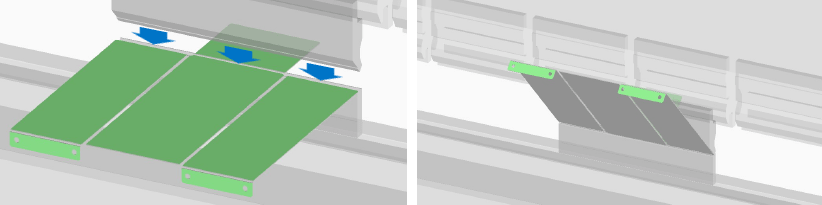Example 2) Model image where die interference occurs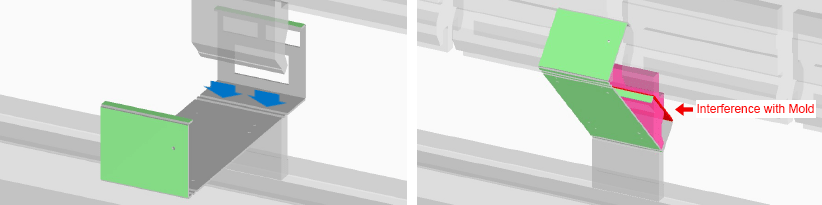• If a warning message is displayed, check the part for any interference using “Check the interference image” and consider changing the shape.
• After taking corrective action, re-upload the 3D model and check the revised quotation results.
• The design guidelines for C-bends and stepped bends (Z-bends) are provided below as more detailed examples. See below.

## Design Guidelines for C-Bends and Stepped Bends (Z-Bends)

To prevent die interference, design models with reference to the standard shapes shown below.

Modeling RulesExample
• When outer dimension A is less than or equal to outer dimension C, set inner dimension B so that it is greater than A.
• Outer dimensions A and C must be at least the minimum bending height (See machining limits: minimum bending height, mentioned above)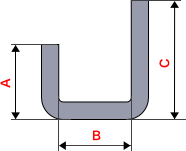• The stepped bend height should be equal to dimension A plus double the plate thickness (t).
• Dimension A must be equal to or greater than the minimum bending height (See machining limits: minimum bending height, mentioned above).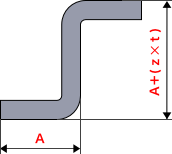However, the meviy platform determines whether it is possible to manufacture the model based upon positive results of the interference analysis. (Examples given below)

Example 3) C-shaped bending interference:　Adjust the size of the channel web in order to clear any interference (the figure on the right shows the analysis image).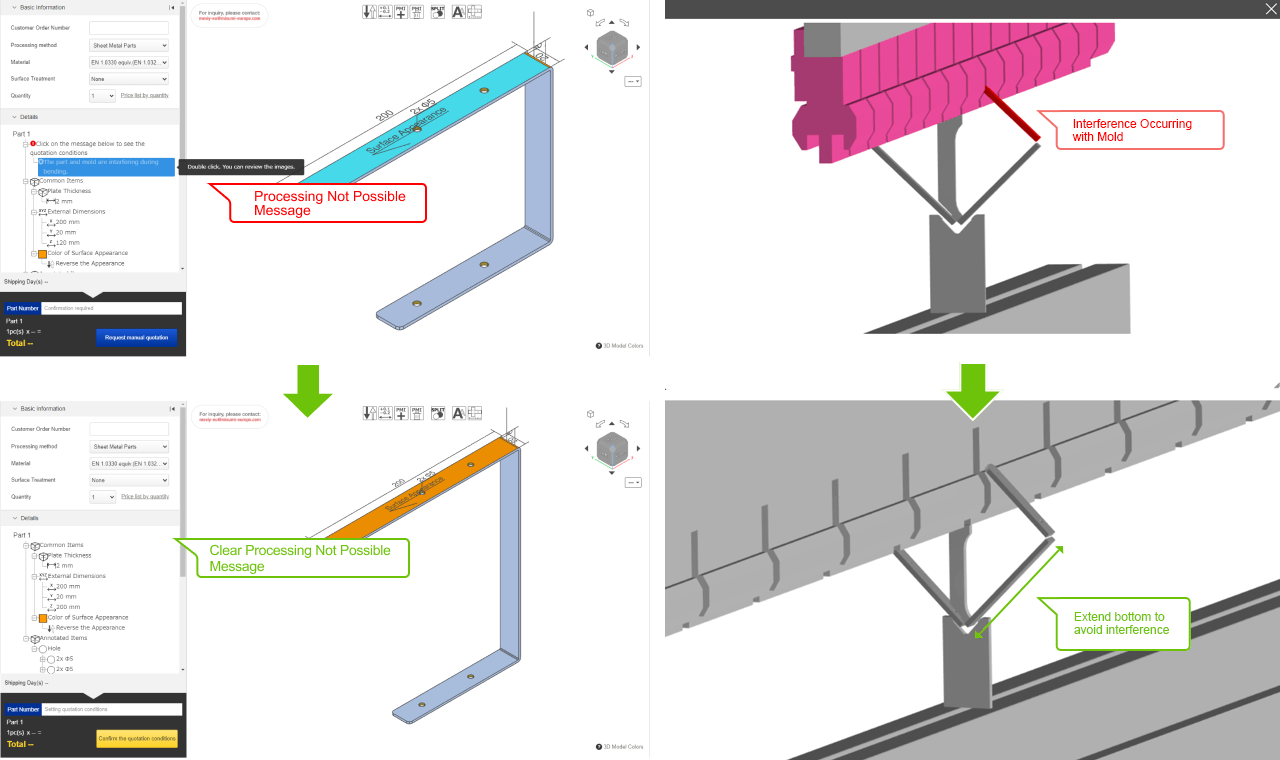Example 4) Stepped bending interference:　Adjust the bending height to clear any interference (the figure on the right shows the analysis image).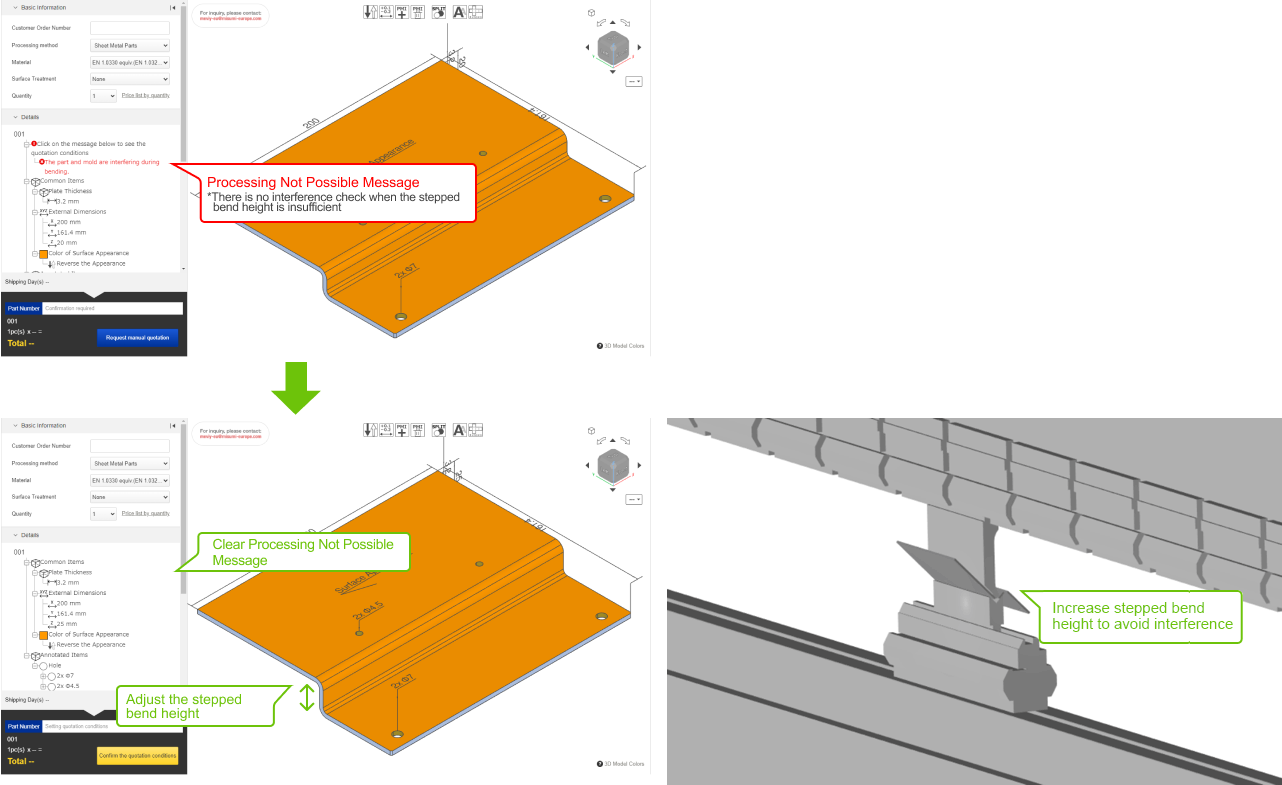## Presence of Edges Parallel to the Bend Line

Any backgauges used in the bending process, along with the edges of parts, are taken into consideration in the interference analysis of the bending process. The angle of the part during the bending process may not be stable if there are no edges parallel to the bend line, creating a risk that the bend may not be made in the correct position. If the below message is displayed, consider changing the shape such that the model includes edges parallel to the bend line.

Example 1) No edges parallel to the bend line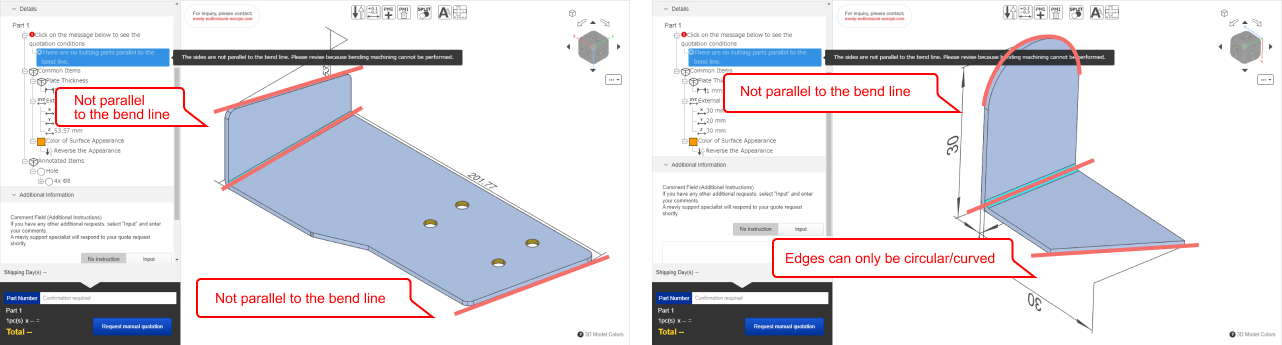Example 2) Edge parallel to the bend line [automatic quoting available]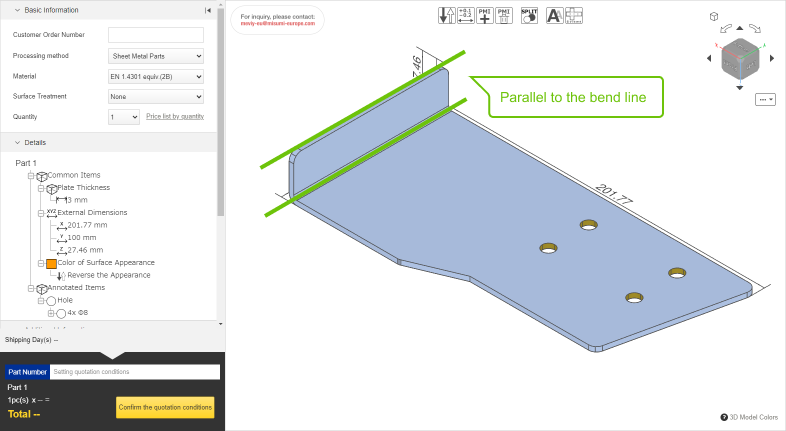## Minimum distance between cutout and bend

• The bending process is carried out by placing the workpiece (to be bent) on a die and punching it from above.
• However, if the shape of the workpiece does not align on both ends of the die, it cannot be bent.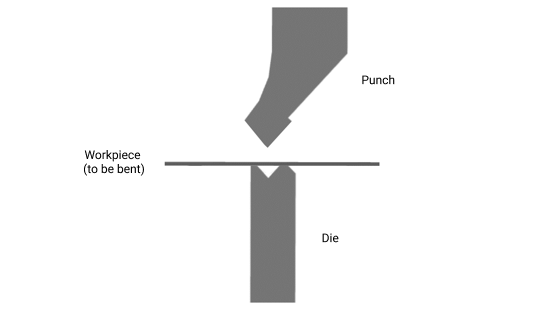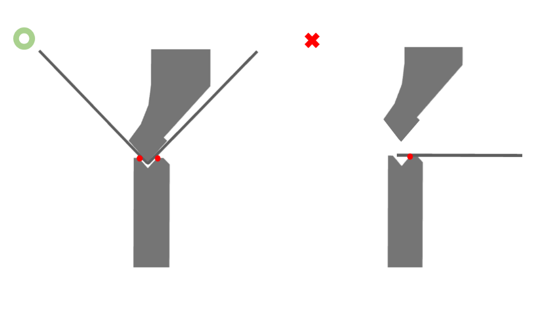• For the minimum distance between the cutout and the bend, we recommend the following.
• Please note that if the value falls below the minimum value, the bending line may shift because part of the workpiece is no longer covered by the bending die.
• The dimensions will be inaccurate, but will be processed as they are.
• In the case of a part that falls below the limit value, it may not be possible to process it. In this case, we will contact you.
•
Modelling rules Location
• For plate thickness t, the limit value of h should be as follows
• 0.8 ≦ t ≦ 2.0, limit value = 5t
• 2.3 ≦ t ≦ 9.0, the limit value = 4t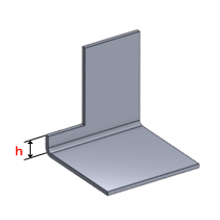• For plate thickness t, the minimum and limit values of b should be as follows
• 0.8 ≦ t ≦2.0, guaranteed value = 2t, limit value = t
• 2.3 ≦ t ≦ 9.0, guaranteed value = none, limit value = 2t